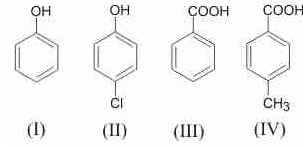Courses

# JEE(MAIN) Chemistry Mock Test - 4

## 30 Questions MCQ Test JEE Main Mock Test Series 2020 & Previous Year Papers | JEE(MAIN) Chemistry Mock Test - 4

Description
This mock test of JEE(MAIN) Chemistry Mock Test - 4 for JEE helps you for every JEE entrance exam. This contains 30 Multiple Choice Questions for JEE JEE(MAIN) Chemistry Mock Test - 4 (mcq) to study with solutions a complete question bank. The solved questions answers in this JEE(MAIN) Chemistry Mock Test - 4 quiz give you a good mix of easy questions and tough questions. JEE students definitely take this JEE(MAIN) Chemistry Mock Test - 4 exercise for a better result in the exam. You can find other JEE(MAIN) Chemistry Mock Test - 4 extra questions, long questions & short questions for JEE on EduRev as well by searching above.
QUESTION: 1

### Phenol can be distinguished from ethyl alcohol by all reagents except

Solution: Phenol reacts with NaOH, doesn't give Bromine water test but alchohol give Bromine water test and don't react with NaOH as pKa of alchohol is more than that of H2O but Na reacts with both phenol and alchohol so Na doesn't distinguish phenol and alchohol. hence D is correct
QUESTION: 2

Solution:
QUESTION: 3

### If R is the Rydberg constant for hydrogen, then wave-number of the second line in the Lyman series is

Solution:

Wave no = 1/λ =R[1/n12 -1/n22]=R[1/12 -1/22]=3R/4.(For Lyman series n1=1)

QUESTION: 4

The ratio of specific charge of a proton and an α-particle is

Solution:
QUESTION: 5
Electrophiles are
Solution:
QUESTION: 6

which of the following hybridisation occurs in the formation of methane molecule?

Solution:
QUESTION: 7
Acetic acid is produced with the help of
Solution:
QUESTION: 8
Structurally, which of the following are similar ions?
Solution:
QUESTION: 9
The molecule having bond order 3 is
Solution:
QUESTION: 10
Tautomerism is exhibited by
Solution:
QUESTION: 11

Which of the following has highest molar conductivity?

Solution:
QUESTION: 12
Which of the following is not a π bonded complex?
Solution:
QUESTION: 13

An aqueous solution of CoCl₂ on addition of excess of concentrated HCl, turns blue due to the formation of

Solution:
QUESTION: 14
Noble gases are
Solution:
QUESTION: 15
Which of the following will act as Lewis base, nucleophilic reagent as well as ligand in complex compound ?
Solution:
QUESTION: 16

Which of the following exhibit geometrical isomerism?

Solution:
QUESTION: 17
Which of the following is obtained when chloral is boiled with NaOH?
Solution:
QUESTION: 18
Effective nuclear charge in case of an ion is equal to-
Solution:
QUESTION: 19
Bakelite is prepared from
Solution:
QUESTION: 20
K, Ca and Li metals may be arranged in the decreasing order of their standard electrode potentials as
Solution:
QUESTION: 21
To 5.85 g of NaCl, one kg of water is added to prepare a solution. What is the strength of NaCl in this colution ? (Molecular weight of NaCl = 58.5)
Solution: 5.85g NaCl = 0.1 mol. As it is present in 1 kg of water, strength = 0.1 molal.
QUESTION: 22

A liquid was mixed with ethanol and a drop of concentrated H2SO4 was added. A compound with a fruity smell was formed. The liquid was

Solution:
QUESTION: 23
Gold number gives
Solution:
QUESTION: 24

Cuprous ore among the following is

Solution:

Cuprite (Cu2O) is the cuprous ore whereas all other ores contain copper as cupric (Cu2+)

QUESTION: 25

Electrolysis of dilute aqueous NaCl solution was carried out by passing 10 milliampere current. The time required to liberate 0.01 mol of H2 gas at the cathode is (1 Faraday = 96500 C mol-1)

Solution:
QUESTION: 26

For a first order reaction A → P, the temperature (T) dependent rate constant (k) was found to follow the equation log k = − (2000) 1/T + 6.0 . The pre-exponential factor A and the activation energy Ea, respectively, are

Solution:
Given, log K = 6 -
$\frac{}{}$
Since, log K = log A -
$\frac{}{}$
So, A =10
${}^{}$
sec
${}^{}$
and Ea =38.3 kJ/mole

QUESTION: 27

Extraction of zinc from zinc blende is achieved by

Solution:
QUESTION: 28

Among the following metal carbonyls, the C ― O bond order is lowest in

Solution:

• Mn+ = 3d54s1 in presence of CO effective configuration = 3d64s0.
Three lone pair for back bonding with vacant orbital of C in CO.

• Fe0 = 3d64s2 in presence of CO effective configuration = 3d8 four lone pair for back bonding with CO.

• Cr0 = 3d54s1
Effective configuration = 3d6. Three lone pair for back bonding with CO.

• V− = 3d44s2 effective configuration = 3d6three lone pair for back bonding with CO. Maximum back bonding is present in Fe(CO)5there for CO bond order is lowest here.

QUESTION: 29

The correct acidity order of the following isSolution:
QUESTION: 30

Among the following, the surfactant that will from micelles in aqueous solution at the lowest molar concentration at ambient conditions is

Solution: